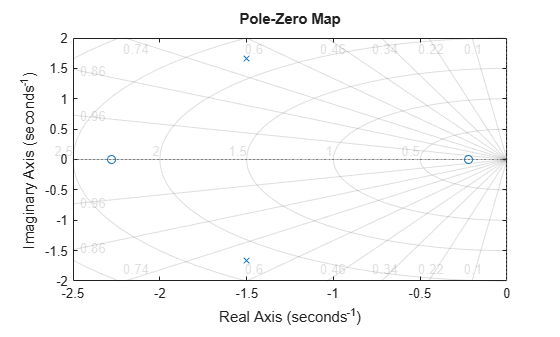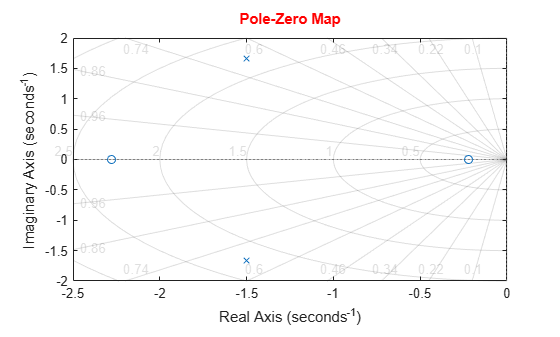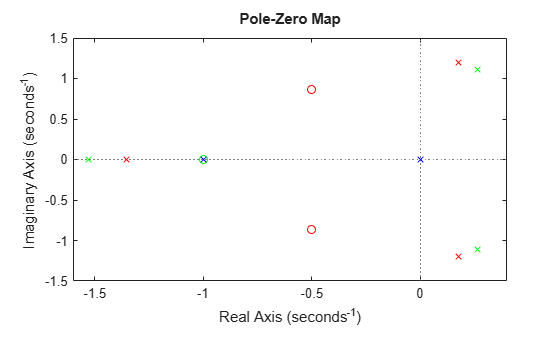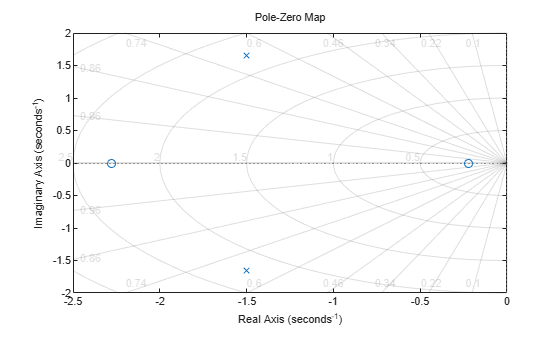# pzplot

Pole-zero plot of dynamic system model with plot customization options

## Syntax

``h = pzplot(sys)``
``pzplot(sys)``
``pzplot(sys1,sys2,...,sysN)``
``pzplot(ax,...)``
``pzplot(...,plotoptions)``

## Description

example

````h = pzplot(sys)` plots the poles and transmission zeros of the dynamic system model `sys` and returns the plot handle `h` to the plot. Use this function to generate a pole-zero map with customizable plot options such as `FreqUnits`, `TimeUnits`, and `IOGrouping`. For more information about using and interpreting pole-zero maps, see `pzmap`.```

example

````pzplot(sys)` plots the poles and transmission zeros of the dynamic system model `sys`. `x` and `o` indicates poles and zeros respectively.```

example

````pzplot(sys1,sys2,...,sysN)` displays the poles and transmission zeros of multiple models on a single plot. You can specify distinct colors for each model individually.```
````pzplot(ax,...)` plots into the axes specified by `ax` instead of the current axis `gca`.```

example

````pzplot(...,plotoptions)` plots the poles and transmission zeros with the options specified in `plotoptions`. For more information on the ways to change properties of your plots, see Ways to Customize Plots.```

## Examples

collapse all

Plot the poles and zeros of the continuous-time system represented by the following transfer function:

`$sys\left(s\right)=\frac{2{s}^{2}+5s+1}{{s}^{2}+3s+5}.$`

```sys = tf([2 5 1],[1 3 5]); h = pzplot(sys); grid on```Turning on the grid displays lines of constant damping ratio (zeta) and lines of constant natural frequency (wn). This system has two real zeros, marked by o on the plot. The system also has a pair of complex poles, marked by x.

Change the color of the plot title. To do so, use the plot handle, `h`.

```p = getoptions(h); p.Title.Color = [1,0,0]; setoptions(h,p);```For this example, load a 3-by-1 array of transfer function models.

```load('tfArrayMargin.mat','sys'); size(sys)```
```3x1 array of transfer functions. Each model has 1 outputs and 1 inputs. ```

Plot the poles and zeros of the model array. Define the colors for each model. For this example, use red for the first model, green for the second and blue for the third model in the array.

`pzplot(sys(:,:,1),'r',sys(:,:,2),'g',sys(:,:,3),'b');`Plot the poles and zeros of the continuous-time system represented by the following transfer function with a custom option set:

`$sys\left(s\right)=\frac{2{s}^{2}+5s+1}{{s}^{2}+3s+5}.$`

Create the custom option set using `pzoptions`.

`plotoptions = pzoptions;`

For this example, specify the grid to be visible.

`plotoptions.Grid = 'on';`

Use the specified options to create a pole-zero map of the transfer function.

`h = pzplot(tf([2 5 1],[1 3 5]),plotoptions);`Turning on the grid displays lines of constant damping ratio (zeta) and lines of constant natural frequency (wn). This system has two real zeros, marked by `o` on the plot. The system also has a pair of complex poles, marked by `x`.

## Input Arguments

collapse all

Dynamic system, specified as a SISO or MIMO dynamic system model, or an array of SISO or MIMO dynamic system models. Dynamic systems that you can use include continuous-time or discrete-time numeric LTI models such as `tf`, `zpk`, or `ss` models.

If `sys` is a generalized state-space model `genss` or an uncertain state-space model `uss`, `pzplot` returns the poles and transmission of the current or nominal value of `sys`. If `sys` is an array of models, `pzplot` plots the poles and zeros of each model in the array on the same diagram.

Axes handle, specified as an axes object. If you do not specify the axes object, then `pzplot` uses the current axes `gca` to plot the poles and zeros of the system.

Pole-zero plot options, specified as an options object. See `pzoptions` for a list of available plot options.

## Output Arguments

collapse all

Pole-zero plot options handle, returned as a scalar. Use `h` to query and modify properties of your pole-zero plot. You can use this handle to customize the plot with the `getoptions` and `setoptions` commands.